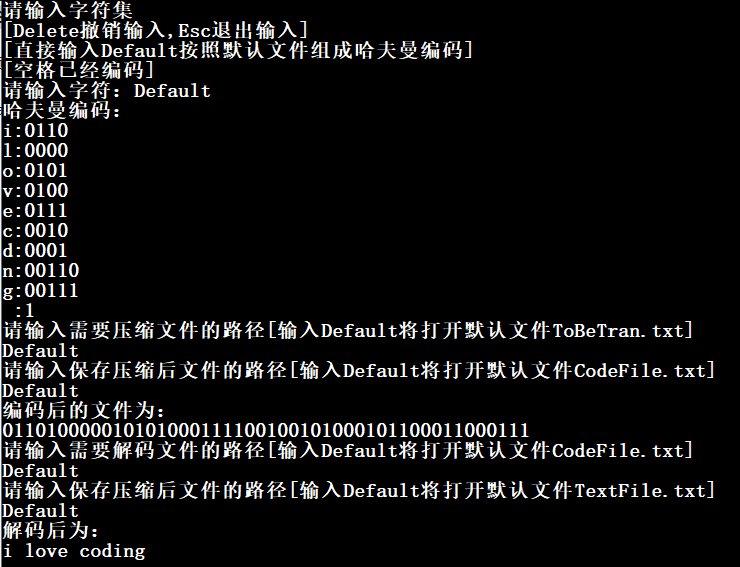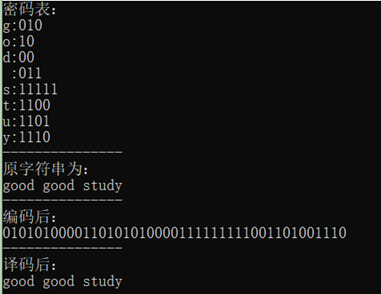• 《数据结构C语言哈夫曼编码译码》由会员分享，可在线阅读，更多相关《数据结构C语言哈夫曼编码译码(16页珍藏版)》请在人人文库网上搜索。1、实训报告题 目： 哈夫曼树编码译码院 系： 信息工程系专 业： 计算机科学...

《数据结构C语言哈夫曼编码译码》由会员分享，可在线阅读，更多相关《数据结构C语言哈夫曼编码译码(16页珍藏版)》请在人人文库网上搜索。
1、实训报告题 目： 哈夫曼树编码译码院 系： 信息工程系专 业： 计算机科学与技术(网络方向)姓 名： 梁展荣 学 号： 指导教师： 赵莹莹 刘欣 日 期： 2013年7月3日 桂林电子科技大学信息科技学院目 录一、设计思想11.1建立哈夫曼树的思想11.2建立哈夫曼编码表21.3对文件进行编码21.4对文件进行解码2二、算法流程图3三、运行结果8四、遇到的问题及解决10五、心得体会13一、设计思想要完成哈夫曼的编码和解码需要首先建立哈夫曼树，之后对所有字符根据权重进行编码，最后再对文件内容进行编码和解码。1.1建立哈夫曼树的思想。首先定义适合哈夫曼树的节点类型，需要定义的有当前节点的字符，当前。
2、节点的左子、右子和父亲指针。在建立哈夫曼树之前还需要对出现的字符和权重进行统计和记录，并且定义一个可以筛选出最小权重的函数。初始化树节点之后开始建立哈夫曼树。先在所有可能出现的字符中筛选出当前权重最小的两个字符，将这两个字符分别作为新节点的左子和右子建立一个小的二叉树，并将两个字符的权重之和赋值给新节点，将新二叉树放入筛选字符中，再将筛选过的两个字符从筛选列表中淘汰掉。依次对列表中剩下的字符进行权重最小的筛选，直到根节点(如果编码表共有N个字符，则2*N-1就为最终根节点)为止，也就是当筛选列表为空的时候，哈夫曼树即建立完成。对于哈夫曼编码树来说，由于哈夫曼编码是前缀码，所以所有要编码的字符最。
3、终都将是这颗树的叶子节点，而其它节点并没有真正的字符意义。即当哈夫曼编码树建立之后，对树的所有叶子节点进行打印可知道是否有字符遗漏或多余。1.2建立哈夫曼编码表。建立编码表时要根据每个出现的字符的权重对建立的哈夫曼树的每个叶子节点进行编码。编码时要从叶子节点出发向根节点进行逆向编码。判断如果当前节点为左子则对其编码0，如果当前节点为右子则对其编码1。以此类推进行编码直到根节点为止。此时的编码是逆向的，所以需要将码值逆向存储。依次对每一个叶子节点进行编码操作，即可得到当前哈夫曼树的编码表。对于码值的逆向存储可以使用栈结构，先将一个码的每一步编码存入栈，再在一个码结束后出栈至空。当然也可以定义一个。
4、字符型数组，将值从后向前存入数组，再将数组有值部分粘贴到新的数组中进行存储。本次采用了后者，因为个人认为为此一步操作建立栈结构不划算，而且前一个设计也已经熟练掌握了栈的方法，此处进行新的尝试会更好。1.3对文件进行编码。首先需要建立一个原始文件，在文件中输入需要编码的内容。之后将文件打开，将其中的内容存储到字符串中以便程序编码调用。开始对需要编码的字符进行编码，将字符逐一读取与刚刚建立的编码表中的每个叶子节点代表的字符进行比较，找出相同的对象，并将当前节点的编码打印到屏幕，并将编码存入到新建的密码文件当中。1.4对文件进行解码。先打开密码文件，将之前编码后得到的密文内容存储到字符串中以便解码调。
5、用。开始对密文的字符串进行解码，树索引从根节点开始走，当密文中的当前字符是0的时候，则索引走向左子节点；当是1的时候，则走向右子节点。以此类推，一直走到叶子节点为止，则当前叶子节点所代表的字符即为前一段密文的解码结果，。再对下一个字符依次从根节点开始解码，如此循环对每一段密文进行解码直到解码结束。将解码打印到屏幕，并将解码结果存入到新的解码文件当中。在解码之前，还应该先确认之前是否建立了哈夫曼树并且是否构建了编码表。不过由于本次将a到z都进行了编码，所以此步省略了，因为编码表是唯一的。需要的时候可以在Encoder 函数中先进行判定。将编码和解码写在了一起，可以在运行时进行选择调用。二、算法流。
6、程图第一步：建立哈夫曼树。图1建立哈夫曼树的算法流程图第二步：构建哈夫曼编码表。图2构建哈夫曼编码表的算法流程图第三步：编码。图3 编码算法流程图第四步：解码。图4 解码算法流程图四、运行结果原文文件：图5 中缀转后缀运行结果图编码图：图6 编码图密文文件：图7 密文文件图解码图：图8 解码图译文文件：图9 译文文件图整体运行图：图10 编码解码整体运行图五、遇到的问题及解决这部分我主要遇到了如下两个问题，其内容与解决方法如下所列：l 第一个问题是权重的筛选部分出现了错误解决办法：一开始对于筛选最小权重的代码编写如下：void SelectMin(HFMT T,int i,int *p1,in。
7、t *p2) int j, min=999;for(j=0;jTj.weight)min=Tj.weight; *p1=j; min=999; for(j=0;jTj.weight&j!=(*p1)min=Tj.weight; *p2=j; 因为权重中最大的就是字符e的权重103，所以为初始值min赋值时觉得999就已经是无限大了。但是后来发现编码不知确，就开始思考是什么问题。发现每次筛选都将会把最小的两个权重进行相加，所以很快就会超过999，编码自然就出现了问题。所以后来将min定义成了long型，并赋值，问题就解决了。l 第二个问题是生成编码表的时候如何将逆向编码正向存储解决办法：对于求编。
8、码的时候，由于是从叶子节点向根顺次而求，所以编码结果将是逆向的。一开始想到的办法是利用栈的结构，将编码依次存入栈中，再在一个字符编码结束时将栈倒空，这样就可以将编码正向存储了。但是又在考虑如果不用栈时候也可以做到。后来想到了strcpy函数对字符数组进行链接。所以就可以定义一个数组，从后向前存储编码，再在一个字符编码结束时将这个数组有值的重新存入新数组中，即可以成为正向编码了。最终实现编码如下：HFCode hfEn(HFMT T) int i,f,c,start;HFCode hc;char *cd;hc=(char *)malloc(N+1)*sizeof(char*); cd=(char。
9、)malloc(N*sizeof(char); cdN-1=0; for(i=0;iN;i+) start=N-1;for(c=i,f=Ti.parent;f!=-1;c=f,f=Tf.parent)if(Tf.left=c) cd-start=0;else cd-start=1;hci=(char *)malloc(N-start)*sizeof(char); strcpy(hci,&cdstart); return hc;六、心得体会通过对本次的编写，使我掌握了哈夫曼编码的特点、存储方法和基本原理，培养了我运用C语言正确编写程序以及调试程序的能力。哈夫曼编码的结构取决于可能出现的字符的个数和其所对应的权值，权值大的编码短，权值小的编码长。这样的结构会利用比较小的空间存储数据。而且，利用树的结构对其编码和对其解码，也是比较规格话，比较方便的。本次编程还运用了结构体，便捷的对树节点和树以及编码表进行定义和调用。并且了解到当求解一个算法时，不是拿到问题就不假思索去做，而应该首先对它有个整体的概念，再一步步对其进行分析。在分析的过程中也应该学会随时总结问题，将遇到的问题融会贯通，以便在将来需要的时候能够应用自如。本次设计中也存在一些一开始不容易解决的问题，但当对算法的进一步分析和对相关信息的查阅，也比较顺利的完成了设计。虽然路途比较艰辛，但奋斗的经历却成为了最宝贵的人生经验。

展开全文• 哈夫曼算法证明 哈夫曼算法是一种贪心算法，我们考虑证明其最优子结构和贪心选择性质： 最优子结构：假设一个树是哈夫曼树，则以其任意节点为根节点的最大子树也是哈夫曼树。 证明：子树的根节点的值是其所有叶子...
哈夫曼算法证明
哈夫曼算法是一种贪心算法，我们考虑证明其最优子结构和贪心选择性质：
最优子结构：假设一个树是哈夫曼树，则以其任意节点为根节点的最大子树也是哈夫曼树。 证明：子树的根节点的值是其所有叶子节点出现权值之和，因此无论子树是什么形式，对子树上方的节点计算WPL2都没有影响。 根据哈夫曼树的定义：WPL最小的二叉树。如果子树不是哈夫曼树，其WPL1就不会是最小，那么整个树的WPL=WPL1+WPL2就不会是最小，这与哈夫曼树的定义相悖，因此子树是哈夫曼树。  贪心选择性质（哈夫曼算法）：每次去掉权值最低的两个节点作为叶子节点形成一颗二叉树，并将其父亲节点放入待选节点中。 证明：对于哈夫曼树我们总可以通过取出两个节点作为叶子节点并将其父亲节点重新作为叶子节点的方式构造，需要证明的是是否每次选择权值最低的节点可以构造成功。 当含有2个及以下的叶子节点的时候，显然正确。 假设当含有小于k个叶子节点的时候哈夫曼算法可以形成哈夫曼树 对于含有k个叶子节点形成的树，设其中权重最小的叶子节点为a,其中深度最深的叶子节点为b，h表示节点的深度，w表示节点的权值。则：

W

P

L

1

=

∑

+

w

a

∗

h

a

+

w

b

∗

h

b

WPL_1=\sum+w_a*h_a+w_b*h_b

交换这两个节点：

W

P

L

2

=

∑

+

w

b

∗

h

a

+

w

a

∗

h

b

WPL_2=\sum+w_b*h_a+w_a*h_b

W

P

L

1

−

W

P

L

2

=

(

w

a

−

w

b

)

∗

h

a

+

(

w

b

−

w

a

)

∗

h

b

=

(

h

b

−

h

a

)

∗

(

w

b

−

w

a

)

WPL_1-WPL_2=(w_a-w_b)*h_a+(w_b-w_a)*h_b=(h_b-h_a)*(w_b-w_a)

而

h

b

−

h

a

h_b-h_a

和

w

b

−

w

a

w_b-w_a

都为正（由a,b节点的性质），所以我们得到结论：对于任何一棵树，将权值小的节点尽可能移动到较深层的节点会使整个树的WPL比较小。
对于k个节点，我们首先取出两个节点，将其合并成一个节点以后我们有k-1个节点，可以使用哈夫曼算法构造。
如果这两个节点不是所有节点中权值最小的两个，则我们总可以通过交换使得构造的树的WPL减小，因此不是哈夫曼树。只有两个节点是所有节点中权值最小的两个时我们无法再降低树的WPL。因为我们总可以构造成功，所以选择权值最小的节点构造的树就是哈夫曼树。证毕。
哈夫曼编码译码程序
#pragma once
#include<iostream>
#include<fstream>
#include<vector>
#include<queue>
#include<cstdio>
#include<string>

using namespace std;

static const int MAXN = 1005;

struct times
{
double weight;//字符的权值
int num;//字符的序号，同时也是他的ASCALL值
times(int _num=0,double _weight=0) :num(_num),weight(_weight){}
friend bool operator < (const times & a,const times & b)
{
return a.weight > b.weight;
}
}p,q;

struct Chara:times
{
int father;//父亲的序号
int lson, rson;//左右儿子的序号
string code;
Chara(int _num = 0, double _weight = 0, int _lson = 0, int _rson = 0, int _father = 0) :times(_num,_weight), lson(_lson), rson(_rson), father(_father)
{
code = "";//没有编码
}
void operator = (const Chara& x)
{
weight = x.weight; num = x.num; father = x.father; lson = x.lson; rson = x.rson;
}
};

struct txt
{
string t;
double weight;
txt(string _t,double _weight):t(_t),weight(_weight){}
};

class HuffmanCode
{
public:
int n=0;//字符的个数
int cur;//当前所在位置
int root;//哈夫曼树根节点
Chara A[MAXN];//顺序表保存哈夫曼树
priority_queue<times> T;//用来构建哈夫曼树
void CreatHuffmanCode(int x, string now)
{
if (A[x].lson != 0)
{
CreatHuffmanCode(A[x].lson, now + "0");
}
if (A[x].rson != 0)
{
CreatHuffmanCode(A[x].rson, now + "1");
}
if (A[x].lson == 0 && A[x].rson == 0)//说明是字符
{
A[x].code = now;
}
}

void _HuffmanCode(int _n,vector<txt>& input)
{
string tmp;
n = _n;
for (int i = 0; i < n; i++)
{
tmp = input[i].t;
A[tmp].num = tmp;
A[tmp].weight = input[i].weight;
T.push(times(tmp, A[tmp].weight));
}
cur = 500;

//构建哈夫曼树
while (T.size() > 1)
{
p = T.top(); T.pop(); q = T.top(); T.pop();
A[cur] = Chara(cur, p.weight + q.weight, p.num, q.num, 0);
A[p.num].father = A[q.num].father = cur;
T.push(times(A[cur])); cur++;
}
T.pop(); root = cur-1;

CreatHuffmanCode(root, "");
}
};

class Huffman
{
int n;
vector<txt> input;
HuffmanCode x;
public:
void _Huffman()
{
string t; double weight; n = 0;
FILE* stream;
freopen_s(&stream,"hfmTree.txt","r",stdin);
while (1)
{
cin >> t;
if (t == "Esc") break;
n++;
cin >> weight;
input.push_back(txt(t, weight));
}
freopen_s(&stream, "CON", "r", stdin);
input.push_back(txt(" ", 10000.0));//给空格很大的权值
n++;
x._HuffmanCode(n, input);
}
Huffman()
{
string t; double weight; n = 0;
cout << "请输入字符集 \n[Delete撤销输入,Esc退出输入]\n[直接输入Default按照默认文件组成哈夫曼编码]\n[空格已经编码]"<< endl;

while(1)
{
cout << "请输入字符：";
cin >> t;
if (n == 0 && t == "Default")//按照默认文件构造哈夫曼树
{
_Huffman();
return;
}
else if (t == "Delete")
{
input.erase(input.end()-1);//删除最后一个输入的字符
n--;
continue;
}
else if (t == "Esc")
{
break;
}
n++;
cout << "请输入" << t << "的权重：";
cin >> weight;
input.push_back(txt(t, weight));
}
FILE* stream;
freopen_s(&stream, "hfmTree.txt", "w", stdout);
for (int i = 0; i < n; i++)
{
cout << input[i].t << " " << input[i].weight << endl;
}
cout << "Esc" << endl;
freopen_s(&stream, "CON", "w", stdout);
input.push_back(txt(" ", 10000.0));//给空格很大的权值
n++;
x._HuffmanCode(n, input);
}
void HuffmanDisplay()
{

cout << "哈夫曼编码：" << endl;
for (int i = 0; i < n; i++)
{
cout << input[i].t << ":" << x.A[input[i].t].code << endl;
}
}
void GenerateCode()//压缩文件
{
cout << "请输入需要压缩文件的路径[输入Default将打开默认文件ToBeTran.txt]" << endl;
string in;

cin >> in;
if (in == "Default")
{
in = "ToBeTran.txt";
}
cout << "请输入保存压缩后文件的路径[输入Default将打开默认文件CodeFile.txt]" << endl;
string out;

cin >> out;
if (out == "Default")
{
out = "CodeFile.txt";
}

ifstream infile(in, ios::in);
ofstream outfile(out, ios::out);

char c;
bool flag = true;
while ((c = infile.get()) != EOF)
{
if (x.A[c].code == "")
{
flag = false;
break;
}
outfile << x.A[c].code;
}

infile.close();
outfile.close();

if (!flag)
{
cout << "压缩失败，文件中出现了字符集中未包含的字符" << endl;
return;
}

//展示压缩的结果:
infile.open(out, ios::in);

string tmp;
infile >> tmp;

infile.close();

cout << "编码后的文件为：" << endl;

for (int i = 0; i < tmp.size(); i++)
{
if (i && i % 50 == 0) cout << endl;
cout << tmp[i];
}
cout << endl;

//将结果放入文件中
outfile.open("CodePrint.txt", ios::out);

for (int i = 0; i < tmp.size(); i++)
{
if (i % 50 == 0) outfile << endl;
outfile << tmp[i];
}

outfile.close();
}
void Decode()
{
cout << "请输入需要解码文件的路径[输入Default将打开默认文件CodeFile.txt]" << endl;
string in;
cin >> in;
if (in == "Default")
{
in = "CodeFile.txt";
}
cout << "请输入保存压缩后文件的路径[输入Default将打开默认文件TextFile.txt]" << endl;
string out;

cin >> out;
if (out == "Default")
{
out = "TextFile.txt";
}

ifstream infile(in, ios::in);

string ss;
infile >> ss;
//cout << "test:" << ss << endl;
int i = 0;
string sss;

while (i < ss.size())
{
int t = x.root;
while ((x.A[t].lson != 0 || x.A[t].rson != 0) && i < ss.size())
{
if (ss[i] == '0') t = x.A[t].lson;
else t = x.A[t].rson;
i++;
}
if (x.A[t].lson == 0 || x.A[t].rson == 0)
{
sss = sss + (char)t;
}
}

infile.close();

cout << "解码后为：" << endl;
cout << sss << endl;

ofstream outfile(out,ios::out);

outfile << sss << endl;

outfile.close();
}
};

测试代码
#include"Huffman.h"

#include<iostream>

using namespace std;

int main()
{

Huffman x;

x.HuffmanDisplay();

x.GenerateCode();

x.Decode();

}


测试结果展开全文• java实现对文件进行哈夫曼编码译码实现。。。。。。。。
• 构建哈夫曼树及哈夫曼编码，输出哈夫曼树及哈夫曼编码，完成编码与译码算法。 （1）掌握树的有关操作算法 （2）熟悉树的基本存储方法 （3）学习利用树求解实际问题
• 希望大家给我指缺点 QQ : 515801610 这个程序能不错的运行 嘿嘿 欢迎大家与我讨论
• 问题描述：利用哈夫曼编码进行信息通讯可以大大提高信道的利用率，缩短信息传输时间，降低传输成本。但是，这要求在发送端通过一个编码系统对待传输数据预先编码；在接受端将传来的数据进行译码。对于双工信道（即...
• 实验名 实 验 方 实 验 实验六 哈夫曼编码译码算法设计与实现 称 案 成绩 实验日 实 验 信息系统设计与仿 实 验 操 2012-04-22 期 室 真室 I 作 实验台 班 级 姓 信工 11-1BF 李煌 实 验 结 34 号 号 名 峰 果 ...
• 哈夫曼编码译码器 利用哈夫曼算法的编码和译码系统，重复地显示并处理以下项目，直到选择退出为止 。
• PAGEPAGE #PAGEPAGE #一、需求分析目前，进行快速远距离通信的主要手段是电报， 即将需... 假设A、B、C、D、的编码分别为00,01,10和11, 则上述7个字符的电文便为“ 0001001010110(”，总 长14位，对方接受时，可按二...

PAGE
PAGE #
PAGE
PAGE #
一、需求分析
目前，进行快速远距离通信的主要手段是电报， 即将需传送的文字转化成由二级制的字符组成的字 符串。例如，假设需传送的电文为“ ABACCDA ”， 它只有4种字符，只需两个字符的串，便可以分辨。 假设A、B、C、D、的编码分别为00,01,10和11, 则上述7个字符的电文便为“ 0001001010110(”，总 长14位，对方接受时，可按二位一分进行译码。
当然，在传送电文时，希望总长尽可能地短。如 果对每个字符设计长度不等的编码，且让电文中出现 次数较多的字符采用尽可能短的编码，则传送电文的 总长便可减少。如果设计 A、B、C、D的编码分别 为0,00,1,01,则上述7个字符的电文可转换成总长为 9的字符串“ 000011010'。但是，这样的电文无法翻 译，例如传送过去的字符串中前4个字符的字串
“ 0000”就可以有很多种译法，或是“ AAAA ”或者 “ BB ”，或者“ ABA ”等。因此，若要设计长短不等 的编码，则必须是任一字符的编码都不是另一个字符 的编码的前缀，这种编码称作前缀编码。
然而，如何进行前缀编码就是利用哈夫曼树来
做，也就有了现在的哈夫曼编码和译码。
二、概要设计
利用哈夫曼树编/译码
(一人建立哈夫曼树
(一人
建立哈夫曼树
(二人 对哈夫曼树进行编码
(三)、输出对应字符的编码
译码过程
主要代码实现:
struct code〃结构体的定义
char a; int w;
int parent; int (child; int rchild;
void creation(code *p,int njnt m); //建立哈夫曼树
〃编码void coding(code *p,int n);
〃编码
void displays code 木pjnt njnt m); 〃输出函数
void translate* char *^hc,code wp,int n);
三、详细设计
x~)＞
x~)＞建立哈夫曩树
宀 o ooo
64623 4 3 6 13图图
646
23 4 3 6 1
3
(一)、对哈夫曼树进行编码主要代码实现:
(一)、对哈夫曼树进行编码
从叶子到根逆for(c=i,f=p[i].pare nt;f!=O;c=f,f=p[f].pare nt) {
从叶子到根逆
if(p[f]」child==c)
{
} else//右孩子编码为'1' 01
} else
//右孩子编码为'1' 01
{
cd[--start]='1：
}
}
(二)、输出对应字符的码
字符
编码
a
110
b
111
c
10
d
0
表
三)、译码过程 要代码实现：
if(strcmp(a,hc[i])==O) //比较两个字符串是否相等,
则输出0
for(c=2* n-1,j=0;a[j]!='\0';j++)
'O'或'1'确定找左孩子或右孩子
{
if(a[j]=='0')〃左孩子
{
c=p[c].lchild;
}
else
//从根出发，按字从跟到叶子顺0 1{
//从根出发，按字
从跟到叶子顺
0 1
c=p[c].rchild;II 右孩子
”的藪字血入雾冲號字臓第if ?数罕运和字母的输图
”的藪字血入雾冲號字臓第if ?数罕运和
字母的输
图
S'
s and S
制⑴?用扎臺个数号:按峯一个鮒字返帶》
H 修新轲人1
也字桃1职臺靜入一个数和=
三、调试分析
数字的输入判断
巒心咲?＜二二二二二二
g新输人
)丄丄i11111
gWfftA.
涣定?Cluu*u*?KLt s
涣定?Cluu*u*?KLt s anil Sr lt,jLTk￡ff \ AiLa.JLiuLsli.ajLiixA it Hi '.buf 1 sulX Vebu.E ^ iLuLlM^ ..
程序是否继续进行的萨卸
判断
lifl
毛否誉滞 '汽術几用者V)否曲辿”
|lBTe^E； -Bjay hey 七? k. ohi t. iiiiAE!
四、用户手册
(一)、首先根据提示输入初始化数据，提示输入一 个数字，请输入一个数a，0
(二)在某一界面结束后，会有“请按回车继续下面 操作"提示，请按提示进行操作，如输入其他数字则 无效，知道输入回车符界面才会跳转。

(三)对界面的操作可以自行选择，在询问是否译码 的时候，请按


展开全文• 设计一个利用哈夫曼算法编码译码系统，重复地显示并处理“要求”中项目，直到选择退出为止。 要求： （1）将权值数据存放在数据文件(文件名为data.txt，位于执行程序的当前目录中) （2）分别采用动态和静态存储...
• 实 验 报 告 2015 / 2016 学年 第二学期 课程名称 数据结构A 实验名称 二叉树的基本操作及哈夫曼编码译码系统的实现 实验时间 2016 年 4 月 14 日 指导单位 计算机科学与技术系 指导教师 骆健 学生姓名 班级学号 ...
• 文章目录实验目的实验设备与环境实验过程及结果分析1. 构造哈夫曼树2. SelectSmall函数3. 哈夫曼编码4. 哈夫曼译码5. 实验结果实验代码main.cppHuffman.h 实验目的 完成Huffman Tree 编码、译码系统的设计 自行设计...


文章目录
实验目的实验设备与环境实验过程及结果分析1. 构造哈夫曼树2. SelectSmall函数3. 哈夫曼编码4. 哈夫曼译码5. 实验结果
实验代码main.cppHuffman.h

实验目的
完成Huffman Tree 编码、译码系统的设计自行设计测试数据自行找一段本文，统计本文中每一个字符出现的频率。理解建立Huffman Tree(编码)过程
实验设备与环境
Dev-C++ 5.11

实验过程及结果分析
1. 构造哈夫曼树
设已知n个结点的权值，根据这些权值构成森林F，森林中的每棵二叉树有且只有一个根结点，权值与之相对应，左右子树为空。 A. 在森林F中选取两颗二叉树根结点的权值最小、次小的树，把它们作为左右子树合成一棵新树，新树权值为左右子树权值的和。 B. 不断重复上述步骤，直到所有树合成一棵树为止。 C. 在合成新树的过程中，每次需要在森林中选取权值最小的两个根结点。因此，引入函数SelectSmall(least,less,i)，在hufftree的前i个向量范围中，找到最小的根结点下标least和次小的根结点下标less。 D. 实现细节中，应注意为树中所有结点预留向量空间，初始化Hufftree，注意只有一个根结点。一共需要执行n - 1次合并根结点。
2. SelectSmall函数
A. 函数SelectSmall在于搜索当前森林中权值最小和次小的两个结点，并将下标分别记录到least、less中。 B. 每一次搜索前先找到当前下标值最小的的单结点（parent域为-1），将下标记录到least中。 C. 寻找最小least下标的过程，可以将将当前least下标的结点的权值与剩下的所有单结点权值作比较，每一次将更小的权值的结点下标赋值给least。 D. 寻找次小less下标的过程同寻找least下标的过程类似，需要在查找过程中加入判断条件，即下标值不等于least，这样查找的结果即为次小下标。
3. 哈夫曼编码
A. 每一个符号的Huffman编码是一个0/1串，因此采用串位储存的方式。本函数采用整数向量存储第i个符号的Huffman编码，并返回该编码串。 B. 第i个符号储存在Huffman树中第i个叶子结点中。为计算它的编码向量，需要向根结点追溯它的结点路径。在路径中左孩子关系记作编码0，右孩子关系记作编码1。由于编码是从叶子结点向根结点逐个读出，次序恰好逆置，因此每个编码数字应该添加在编码向量的首部，这样才能得到编码向量的正确次序。
4. 哈夫曼译码
A. 当已知编码文件，需要还原信息符号串时，需要依据编码时的原Huffman树进行译码。函数Decode(vector &source)中参数source是编码文件(0/1向量),返回值是原信息符号串。 B. 每个符号的译码工作都是从Huffman树的根向叶子结点的下行过程。逢0向左孩子下行，逢1向右孩子下行。当下行遇到叶子结点时，该叶子中的data域的值就是译码符号。 C. 每找到一个叶子结点并取其data域的值，再将当前结点再次置为根结点，直到找到最后一个叶子结点的data值。
5. 实验结果
原字符串以“good good study”为例子，实验结果如下：
01234567891011121314datagodstuyweight0.130.270.20.130.070.070.070.070.140.140.260.280.460.551.0parent101312108899111112131414-1lchild-1-1-1-1-1-1-1-146082112rchild-1-1-1-1-1-1-1-15739101113实验代码
main.cpp
#include <iostream>
#include "Huffman.h"

/* run this program using the console pauser or add your own getch, system("pause") or input loop */

int main(int argc, char** argv) {
char ss[]="good good study";
//char ss[]="BACECDC";
/*char data[] = {'w','h','e','r',' ','t','i','s','a','w','l',',','y'};
double weight[] = {0.03,0.08,0.17,0.08,0.19,0.06,0.08,0.06,0.08,0.06,0.06,0.03,0.03};
*/
char data[] = {'g','o','d',' ','s','t','u','y'};
double weight[] = {0.13,0.27,0.20,0.13,0.07,0.07,0.07,0.07};
int len=strlen(data);
HuffmanNode a[len] ;

for(int i=0;i<len;i++)
{
a[i].data = data[i];
a[i].weight = weight[i];
a[i].lchild = a[i].rchild = -1;
a[i].parent = -1;
}
vector<HuffmanNode> t(a,a+len);
HuffmanTree hu(t);
//print(hu.hufftree,2*len-1);
cout<<"密码表："<<endl;
for(int j=0;j<len-1;j++)
{
vector<int>code = hu.GetCode(j);
cout<<hu.hufftree[j].data<<":";
for(int i=0;i<code.size();i++)
cout<<code[i];
cout<<endl;
}
cout<<"---------------"<<endl;
cout<<"原字符串为："<<endl<<ss<<endl;
cout<<"---------------"<<endl;
cout<<"编码后："<<endl;
vector<int>encode;
for(int i=0;i<strlen(ss);i++)
{
int j;
for(j=0;j<hu.hufftree.size();j++)
if(ss[i] == hu.hufftree[j].data)
break;
vector<int>code = hu.GetCode(j);
encode.insert(encode.end(),code.begin(),code.end());
}
for(int i=0;i<encode.size();i++)
cout<<encode[i];
cout<<endl<<"---------------"<<endl;
cout<<"译码后："<<endl<<hu.Decode(encode);
return 0;
}

Huffman.h
#include<bits/stdc++.h>
using namespace std;

struct HuffmanNode
{
char data;	            // 待编码的符号
double weight;	            // 符号出现的频率
int parent, lchild, rchild;       // 父结点、左, 右孩子结点的位置
};

class HuffmanTree
{
//vector<HuffmanNode> hufftree;  // 树中所有结点的存储空间
int n;			           // 叶子结点数
void SelectSmall(int &least,int &less,int i);
public:
vector<HuffmanNode> hufftree;
HuffmanTree(vector<HuffmanNode> &leafs);
vector<int> GetCode( int i );
string Decode(vector<int> &source);
//    string Encode()
};

HuffmanTree::HuffmanTree(vector<HuffmanNode> &leafs)
{
n = leafs.size();
hufftree.resize(2*n-1);
for(int i=0;i<n;i++)
{
hufftree[i].data = leafs[i].data;
hufftree[i].weight = leafs[i].weight;
hufftree[i].parent = hufftree[i].lchild
= hufftree[i].rchild
= -1;
}
for(int i=n;i<2*n-1;i++)
{
int least,less;
SelectSmall(least,less,i);
hufftree[least].parent = hufftree[less].parent = i;
hufftree[i].parent = -1;
hufftree[i].lchild = least;
hufftree[i].rchild = less;
hufftree[i].weight = hufftree[least].weight
+ hufftree[less].weight;
}
}
//--------------------------
void HuffmanTree::SelectSmall(int &least,int &less,int i)
{
for (int j = 0; j < i; j++)
if (hufftree[j].parent == -1)
{
least = j;
break;
}
for (int j = 0; j < i; j++)
if (hufftree[j].parent == -1 && hufftree[least].weight > hufftree[j].weight)
least = j;
for (int j = 0; j < i; j++)
if (hufftree[j].parent == -1&&j!=least)
{
less = j;
break;
}
for (int j = 0; j < i; j++)
if (hufftree[j].parent == -1 && hufftree[less].weight > hufftree[j].weight&&j != least)
less = j;
}
//--------------------------
vector<int> HuffmanTree::GetCode( int i )
{
vector<int>code;
int p = i;
int parent = hufftree[i].parent;
while(parent!= -1)
{
if(hufftree[parent].lchild == p)
code.insert(code.begin(),0);
else
code.insert(code.begin(),1);
p = parent;
parent = hufftree[parent].parent;
//cout<<code[i];
}
return code;
}
//--------------------------
string HuffmanTree::Decode(vector<int> &source)
{
string target ="";
int root = hufftree.size() -1;
int p = root;
for(int i=0;i<source.size();i++)
{
if(source[i] == 0)
p = hufftree[p].lchild;
else
p = hufftree[p].rchild;
if(hufftree[p].lchild == -1
&& hufftree[p].rchild == -1)
{
target = target + hufftree[p].data;
p = root;
}
}
return target;
}

void print(vector<HuffmanNode> hT,int n)
{
cout << "index weight parent lChild rChild" << endl;
cout << left;    // 左对齐输出
for (int i = 0; i < n; ++i)
{
cout << setw(5) << i << " ";
cout << setw(6) << hT[i].weight << " ";
cout << setw(6) << hT[i].parent << " ";
cout << setw(6) << hT[i].lchild << " ";
cout << setw(6) << hT[i].rchild << endl;
}
}

展开全文数据结构
• 一、实验目的和要求 目的：1、掌握二叉链表上实现二叉树基本操作...3、理解哈夫曼树的构造算法，学习设计哈夫曼编码译码系统 要求：能成功演示二叉树的有关算法，运算完毕后能成功释放二叉树所有结点占用的系统类存。
• (2)编码：利用建好的哈夫曼树生成哈夫曼编码； (3)输出编码； (4)设字符集及频度如下表： 字符 空格 A B C D E F G H I J K L M 频度 186 64 13 22 32 103 21 15 47 57 1 5 32 20 字符 N O P Q R...
• 程序设计任务： 设计一个程序，实现哈夫曼编码译码的生成算法。基本要求：输入字符集大小n，以及n个字符和n个权值；构造哈夫曼树，产生每个字符的Huffman编码, 打印之；输入电文，将其翻译成比特流, 打印之；输入...
• 哈夫曼编码译码器实验报告，内有源代码，vc++6.0写的
• （3）输入一个待解压缩的压缩文件名，并利用相应的赫夫曼树将编码序列译码（解码）。（4）显示指定的编码文件和文本文件。（5）把赫夫曼编码用二进制位紧缩到一个变量中，利用位运算进行真正的数据压缩，并求压缩比...CC
• 根据哈夫曼编码算法，来实现将字符编码为相应的01编码，也可以将01编码转化为相应的字符编码。 此代码在实现时还要建立若干的文件： 1）建立一个存放要编码的字符文件.in。 2）建立一个存放编码后存放01编码的文件...
• 哈夫曼编码译码系统的实现，主要包含三部分： 1、创建哈夫曼树 2、编码函数 3、译码函数 编写代码时为了方便，在这里混用了c++的输入输出流。主体用c语言实现。 下面是代码部分： 1、头文件，以及储存结构： #...c++ 数据结构
• 该楼层疑似违规已被系统折叠隐藏此楼查看此楼#include#definemaxvalue10000/*定义最大权值常量*/#definemaxnodenumber100/*定义结点最大数目常量*/#definemaxbit100/*定义哈夫曼编码的最大长度*/typedefstruct...
• 哈夫曼编码译码 包括默认编码 和 自定义编码 数据结构课程设计 一、题目: 哈夫曼编码/译码的设计与实现 二、目的与要求 1、目的： 通过布置具有一定难度的实际程序设计项目，使学生进一步理解和掌握课堂上所学...
• 源于一次实验课，要求实现哈夫曼树、哈夫曼编码译码；我就直接贴实验要求和代码实现了。 注：因为当时时间有限，故其实该代码还有优化空间，且输出文件是0/1字符串文本（UTF-8）并不是ASCII码编码文件，计算压缩率...
• 问题描述和求解方法：首先根据给定的n个字符的权值构造哈夫曼树。通过遍历此二叉树完成各字符的哈夫曼编码，另输入一组‘0’、‘1’代码构成的报文将其翻译成对应的字符信息。
• 哈夫曼编码译码器 仅供学习和参考，这份代码的算法和文件操作都参考了许多文章。 仅供学习和参考，这份代码的算法和文件操作都参考了许多文章。 仅供学习和参考，这份代码的算法和文件操作都参考了许多文章。 1. ...java 数据结构
• 求大神帮忙写个哈夫曼编码译码器用c语言 设计内容： 设计一个利用哈夫曼算法的编码和译码系统，重复地显示并处理以下业务，直到选择退出为止。 (1) 初始化：键盘输入n个字符和n个权值，建立哈夫曼树(n&amp;gt...c语言
• 哈夫曼编码/译码系统。 要求： 能成功演示二叉树的有关运算， 运算完毕后能成功释放二叉树所有结点占用的系统内存。 程序一：二叉树的创建以及基本运算 main.cpp #include"BTree.h" #include int main...数据结构 二叉树 编码
• 1.掌握二叉树的二叉链表存储表示及遍历操作实现方法。 //完成二叉树的创建，先序遍历，中序遍历，后续遍历 #include&lt;iostream&gt; #include&lt;cstring&gt; using namespace std; typedef ......